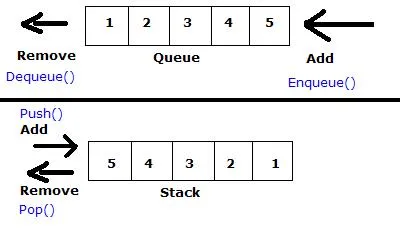# Programming - C Stack-Queue Exercise using Dynamic ArraysHello again, in today's post I would like to show you a Stack-Queue Exercise that I had to do in my University and it's Solution. We had to do almost the same Exercise using Dynamic Arrays and Linked Lists and I will post them separately. As always, this is my Code and someone else could have done it in another way that could be easier or more difficult then my way. We had only like 2 Weeks for both Exercises and the Exercises were also changed in between, cause some Students had problems with some parts of them. So, without further do. Let's get started!

Suppose we have 2 Ferry Boats called S and Q, where S works like a Stack and Q works like a Queue. There will be Cars that choose in which one of those boats they want to go (we will read from a file).

Those 2 Boats will be defined as structs that contain:

• the capacity (to check if the Boats are full)
• the sailing number (for writing into files)
• an dynamic car_id array
• top index for Stack or front and rear index for Queue

The Cars will be a part of a text file called cars.txt (we will get the name as input) and this file will contain the car_id and the boat preference like this:

## cars.txt:

ZZA9896 S

MIN5689 Q

NHI9098 S

KNM4545 Q

IPO8987 Q

The Programm will:

1. get the capacity of each boat and the file name as user input
2. initialize the boats,
3. start reading from the file and then manage the sailings of both boats by filling them until they are full
4. when a boat is full we will start a "sailing" by writing the car_id's into a new file with name s0,s1,... if Stack or q0,q1,... if Queue and then reset the boat
5. the reading loop will stop when there are no more cars in the file
6. then we will check if there are some remainders in the Boats to write them in a "sailing" file aswell

The Stack-Queue Functions need to be Functions that are called from the main function!

# Solution:

``#include <stdio.h>``
``#include <stdlib.h>``
``#include <string.h>``

``// Stack struct``
``typedef struct Stack{``
`` int sail_num;``
`` int capacity;``
`` int top;``
`` char **car_id;``
``}Stack;``

``// Queue struct``
``typedef struct Queue{``
`` int sail_num;``
`` int capacity;``
`` int front;``
`` int rear;``
`` char **car_id;      ``
``}Queue;``

``// Function Declarations``
``void push(Stack *S, char *x);``
``void pop(Stack *S, char *car_id);``
``void StacktoFile(Stack *S);``
``void add(Queue *Q, char *x);``
``void delete(Queue *Q, char *car_id);``
``void QueuetoFile(Queue *Q);``

``int main(){``
`` // Boat variables``
`` Stack S;``
`` Queue Q;``
`` char input_file;``
`` int i;``
`` FILE *fp;``
`` // temp file reading buffers``
`` char car_id;``
`` char ship;``

`` // read capacity values``
`` printf("Capacity of S: "); ``
`` scanf("%d", &S.capacity);``
`` printf("Capacity of Q: ");``
`` scanf("%d", &Q.capacity);``

`` // read file name``
`` printf("Input File: ");``
`` scanf("%19s", input_file);``

`` // open file for reading``
`` if(!(fp=fopen(input_file, "r"))){ ``
``     perror("fopen");``
``     return 1;``
`` } ``

`` // initialization of Stack``
`` S.car_id=(char**)malloc(S.capacity*sizeof(char*)); // lines``
`` for(i=0;i<S.capacity;i++){``
``     S.car_id[i]=(char*)malloc(10*sizeof(char)); // rows``
`` }   ``
`` S.top=-1;``
`` S.sail_num=0;``

`` // initialization of Queue``
`` Q.car_id=(char**)malloc(Q.capacity*sizeof(char*)); // lines``
`` for(i=0;i<Q.capacity;i++){``
``     Q.car_id[i]=(char*)malloc(10*sizeof(char)); // rows``
`` }``
`` Q.front=Q.rear=-1;``
`` Q.sail_num=0;``

`` // loop for reading from file and writing into new ones``
`` while(fscanf(fp, "%9s %c", car_id, &ship) != EOF){ ``
``     // ship S Code``
``     if(ship=='S'){``
``         push(&S, car_id);``
``         // if full write to File``
``         if(S.top == S.capacity - 1){``
``             StacktoFile(&S);``
``         }           ``
``     }``
``     // ship Q Code``
``     else{``
``         add(&Q, car_id);``
``         // if full write to File``
``         if(Q.rear == Q.capacity -1){``
``             QueuetoFile(&Q);``
``         }``
``     }``
`` }``

`` // check if boats are left``
`` if(S.top!=-1) StacktoFile(&S);``
`` if(Q.front!=-1) QueuetoFile(&Q);``
`` fclose(fp);``
`` return 0;   ``
``}``

``// Stack Functions``
``void push(Stack *S, char x[]){``
`` if(S->top < (S->capacity - 1)){``
``     S->top++;``
``     strcpy(S->car_id[S->top], x);``
`` }``
`` // if full write to File``
`` else{``
``     StacktoFile(S);``
`` }``
``}``
``void pop(Stack *S, char *car_id){``
`` if(S->top==-1){``
``     printf("Stack is empty!\n");``
`` }``
`` else{``
``     strcpy(car_id, S->car_id[S->top]);``
``     S->top--;``
`` }``
``}``
``void StacktoFile(Stack *S){``
`` FILE*fp;``
`` int i;``
`` char output_file;``
`` char buffer;``
`` char car_id;``
`` // convert from int to string``
`` itoa(S->sail_num, buffer, 10);``
`` strcpy(output_file, "s");``
`` strcat(output_file, buffer);``
`` strcat(output_file, ".txt");``
`` // write into file``
`` fp=fopen(output_file, "w");``
`` while(S->top>-1){``
``         pop(S, car_id);``
``         fprintf(fp, "%s\n", car_id);``
`` }``
`` S->sail_num++;``
`` fclose(fp);``
``}``

``// Queue Functions``
``void add(Queue *Q, char x[]){``
`` // if full write to File``
`` if(Q->rear == Q->capacity -1){``
``     QueuetoFile(Q);``
``     return;``
`` }``
`` else if(Q->front == -1){``
``     Q->front=Q->rear=0;``
`` }``
`` else{``
``     Q->rear++;``
`` }``
`` strcpy(Q->car_id[Q->rear], x);``
``}``
``void delete(Queue *Q, char *car_id){``
`` if(Q->front==-1){``
``     printf("Queue is Empty!\n");``
`` }``
`` else{``
``     if(Q->front==Q->rear){``
``         strcpy(car_id, Q->car_id[Q->front]);``
``         Q->front=Q->rear=-1;``
``     }``
``     else{``
``         strcpy(car_id, Q->car_id[Q->front]);``
``         Q->front++;``
``     }``
`` }``
``}``
``void QueuetoFile(Queue *Q){``
`` FILE*fp;``
`` int i;``
`` char output_file;``
`` char buffer;``
`` char car_id;``
`` // convert from int to string``
`` itoa(Q->sail_num, buffer, 10);``
`` strcpy(output_file, "q");``
`` strcat(output_file, buffer);``
`` strcat(output_file, ".txt");``
`` // write into file``
`` fp=fopen(output_file, "w");``
`` while(Q->front!=-1){``
``     delete(Q, car_id);``
``     fprintf(fp, "%s\n", car_id);``
`` }``
`` Q->sail_num++;``
`` fclose(fp);``
``}``

That was the end of today's post! Hope you enjoyed it!